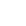# Analytic Resources

We regularly use a number of analytic tools for computational neurosciences in our research, including brain network analysis and neural simulations. These codes are open source software tools in Python and can be found on the lab's GitHub account.  All routines require NumPy, SciPy and Matplotlib to be installed; some functions optionally use Weave for embedded C++ code.

The implemented routines provide functionality to

• construct functional networks using different statistical dependence measures
• calculate graph metrics
• perform various forms of absolute and relative network thresholding
• construct random graphs
• visualize networks in 2D or 3D
• render the spatial embedding of networks in three-dimensional brain surfaces
• simulate brain activity using a biological neural population model